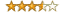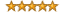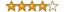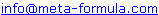# Metastock Explorer Formulas Click here to go back to Metastock Formula Index

Before you begin, if you haven't read the "The Search for the Holy Grail & the Perfect Indicator" click here and scroll halfway down the page to view it now.

Also, click here to discover the amazingly simple secret to master Metastock step-by-step.

Bottom Reversal
Close Above Median Price
High
er Closes
High Volume
Equis' Price & VolumeStocks Closing Above 60 Day HighsLong Term Up Trend & Short Term Re-Entry Signals
Moving Average Crossover - Bullish

RSC Exploration CodingTurtle's Traders Entry SystemReady To Use Breakout Formulas - Collection #1

Contained in this PDF is a small collection of breakout formula that we have found useful. Please feel free to cut and paste them and use them in your own explorers. Click Here to download collection #1

Bottom Reversal - Metastock Formula

These are a collection of bottom signals.
The search returns 1 for Ok and 0 for not ok.

Col A: CLOSE
Col B: EngulfingBull()
Col C: MorningDojiStar()
Col D: MorningStar()
Col E: WhiteSoldiers()

Close Above Median Price - Metastock Formula
by The Strategic Electronic Day Trader.

This exploration is designed to find those stocks where the close is above the median price over the past five days. It matches the steps in Dels book "The Strategic Electronic Day Trader".

Col A: CLOSE - MP()
Col B: (Ref(CLOSE,-1))-(Ref( MP() ,-1))
Col C: (Ref(CLOSE,-2))-(Ref( MP() ,-2))
Col D: (Ref(CLOSE,-3))-(Ref( MP() ,-3))
Col E: (Ref(CLOSE,-4))-(Ref( MP() ,-4))

Filter: colA>=0 AND colB>=0 AND colC>=0 AND colD>=0 AND colE>=0

The filter in the exploration only shows those stiocks that have the strongest bullish bias over all 5 days. By removing the filter all stocks will be shown. Ranking the first colum will then allow you to estaboish the overall score for each stock.

Higher Closes - Metastock Formula

Shows stocks which have closed higher on successive days.

Col A: CLOSE
Col A: CLOSE -1
Col A: CLOSE -2

Filter: When(colA,>,colB) AND When(colB,>,colC)

High Volume - Metastock Formula

Displays those where volume is above the 100 day moving average.
The search returns 1 for Ok and 0 for not ok.

Col A: VOLUME
Col A: Mov(VOLUME,100,EXPONENTIAL)
Col A: ((VOLUME - Mov(VOLUME,100,EXPONENTIAL)) / Mov(VOLUME,100,EXPONENTIAL)) * 100

Filter: When(colA,>,colB)

Equis' Price & Volume - Metastock FormulaBy Equis International Metastock Explorers

Col A: Close CLOSE
Col B: Previous Ref(CLOSE,-1)
Col C: % change ROC(CLOSE,1,percent)
Col D: Volume VOLUME
Col E: M.A. Mov(VOLUME,50,EXPONENTIAL)
Col F: % above ((VOLUME - Mov(VOLUME,50,EXPONENTIAL)) /
Mov(VOLUME,50,EXPONENTIAL)) * 100

Filter colC >= 5 AND colD >= colE*1.5

Long Term Up Trend with Short Term Re-Entry Signals
By Hitendra Vasudeo - www.stockmechanics.com

a:=(Cross( Mov(CLOSE , 2, E)  , Mov(CLOSE ,8 ,S )  ) AND
CLOSE > Mov(CLOSE ,200 ,E ) AND
CLOSE > Mov(CLOSE , 200, S) AND
Mov(CLOSE ,200 ,E ) > Mov(CLOSE ,200 ,S )) OR
Cross( Mov(CLOSE ,200 ,E )  ,  Mov(CLOSE ,200 ,S ) ) ;
b:=  Cross( Mov(CLOSE ,200 ,S )  ,CLOSE ) OR
Cross(Mov(CLOSE ,200 ,E ) , CLOSE);
state > Ref(state,-1)

a:=Cross( Mov(CLOSE , 2, E)  , Mov(CLOSE ,8 ,S )  ) AND
CLOSE > Mov(CLOSE ,200 ,E ) AND
CLOSE > Mov(CLOSE , 200, S) AND
Mov(CLOSE ,200 ,E ) > Mov(CLOSE ,200 ,S );
b:=  Cross( Mov(CLOSE ,200 ,S )  ,CLOSE ) OR Cross(Mov(CLOSE ,200 ,E ) , CLOSE);
state < Ref(state,-1)

Highlights Profit Booking

(HHV(HIGH ,13 ) > Ref( HHV(HIGH ,13 ),-13) AND
HHV( RSI(CLOSE ,13 )  ,13) < Ref(HHV( RSI(CLOSE ,13 )  ,13 ) ,-13)) AND
CLOSE > Mov(CLOSE ,200 ,S ) AND
CLOSE > Mov(CLOSE ,200 ,E ) AND
Mov(CLOSE ,200 ,E )>Mov(CLOSE ,200 ,S )AND
(HHV(HIGH , 4)= HHV(HIGH ,90))

MACD Crossover Buy Signal - Metastock Formula

Shows those stocks where an MACD crossover has been signalled.The search returns 1 for Ok and 0 for not ok.

Col A: CLOSE
Col B: MACD()
Col C: Ref(MACD(),-1)
Col D: Mov(MACD(),9,EXPONENTIAL)
Col E: Ref(Mov(MACD(),9,EXPONENTIAL),-1)
Col F: ((MACD() - Mov(MACD(),9,EXPONENTIAL)) /Mov(MACD(),9,EXPONENTIAL)) * 100

Filter: Cross( MACD(), Mov(MACD(),9,EXPONENTIAL))

Stocks Closing Above 60 Day High
- Metastock Formula
By Rajat BoseTo find the securities that have closed above their high today (the last
trading day in the database) for the first time, I have written this
MetaStock Explorer.

ColA: {Close) C
ColB: {Previous 60-day High} Ref(HHV(H,60), -1)
ColC: {Current 60-day High} HHV(H,60)
ColD: {Volume} V
Filter: (colA>colB) AND (Ref(C,-1)<Ref(HHV(H,60), -1)) AND
(H=HHV(H,60))

This formula does two things:

1) It lists only those securities which have met the required conditions
only on the last trading day.
2) The new 60-day high must have taken place only on the last trading day.

Moving Average Crossover - Bullish - Metastock Formula

This is a10 and 30 day moving average crossover search. Results close to 0 pinpoint the crossover.

Col A: CLOSE
Col A: Mov(CLOSE,30,EXPONENTIAL)
Col A: ((CLOSE-Mov(CLOSE,30,EXPONENTIAL)) /Mov(CLOSE,30,EXPONENTIAL)) * 100
Col A: ((CLOSE-Mov(CLOSE,10,EXPONENTIAL)) /Mov(CLOSE,10,EXPONENTIAL)) * 100

Filter: When(colA > colB)

RSC Exploration Coding - Metastock Formulaby MetaStock Programming Study Guide

Col A: % MA RSC ROC(Mov((C/P),13,S),1,%)

Turtle's Traders Entry System - Metastock Formulaby MetaStock Programming Study Guide

Col A: Close C
Col B: Previous Ref(C,-1)
Col C: ROC ROC(C,1,%)
Col D: Avg T/O Mov(C,21,S)*Mov(V,21,S)

Filter: H>=Ref(H,-1) AND H>=Ref(H,-2) AND H>=Ref(H,-3)
AND H>=Ref(H,-4) AND Mov(C,13,E)>
Ref(Mov(C,13,E),-1) AND
Trough(1,L,4)>Trough(2,L,4)AND C>Mov(C,180,E) AND
O>Ref(C,-1) AND L > Ref(H,-5)

Please email to